# Научный форум dxdy

Математика, Физика, Computer Science, Machine Learning, LaTeX, Механика и Техника, Химия,
Биология и Медицина, Экономика и Финансовая Математика, Гуманитарные науки

 Печатать страницу | Печатать всю тему Пред. тема | След. тема

 Re: Al Zimmermann - Sums and Products21/02/10
1594
Екатеринбург
 Herbert Kociemba в сообщении #1082546 писал(а):Then there seems to be an error in your program logic.Наверно расчет текущего рекорда дает неверный результат из-за округления результатов.Re: Al Zimmermann - Sums and Products24/11/10
48
 MaksSok.95Цитата:Программа conpp выдает решение {0,1,3} (для работы под виндами я заменил isort на qsort и durand на dlarnv)Я пробовал скомпилировать эту программу с G77: qsort он принял, а вот dlarnv он понятия не имеет что это такое. Please help!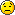Re: Al Zimmermann - Sums and Products21/02/10
1594
Екатеринбург
 dimkadimon в сообщении #1080827 писал(а): Все таки хочется удивить лидеров.Как получить 30 баллов в общем понятно. Но не очень хочется тратить время на преодоление технических трудностей.А вот как приготовить новогодний сюрприз для лидеров нет никаких идей.Re: Al Zimmermann - Sums and Products13/12/15

91
 Последний раз редактировалось MaksSok.95 17.12.2015, 11:30, всего редактировалось 2 раз(а). Vitaly12 в сообщении #1082862 писал(а):MaksSok.95Цитата:Программа conpp выдает решение {0,1,3} (для работы под виндами я заменил isort на qsort и durand на dlarnv)Я пробовал скомпилировать эту программу с G77: qsort он принял, а вот dlarnv он понятия не имеет что это такое. Please help!Я использую Microsoft Visual Studio Enterprise 2015 + Intel Parallel Studio XE 2016. dlarnv - это качественный генератор случайных чисел из пакета Intel MKL.-- 17.12.2015, 10:56 --Pavlovsky в сообщении #1082898 писал(а):dimkadimon в сообщении #1080827 писал(а): Все таки хочется удивить лидеров.Как получить 30 баллов в общем понятно. Но не очень хочется тратить время на преодоление технических трудностей.А вот как приготовить новогодний сюрприз для лидеров нет никаких идей.Наверное, никак: у меня для n=23 решение получилось мгновенно (395). Правда, не знаю, оптимально ли оно.-- 17.12.2015, 11:30 --Вот модифицированный кол: код: [ скачать ] [ спрятать ]код: [ скачать ] [ показать ]Используется синтаксис Fortran cccccccccccccccccccccccccccccccccccccccccccccccccccccccccccccccccccccccc c c        Program to construct Golomb rulers from projective planes c cccccccccccccccccccccccccccccccccccccccccccccccccccccccccccccccccccccccc c c                  IBM SOFTWARE DISCLAIMER c c   conpp.f (version 1.1) c   Copyright (1998,1986) c   International Business Machines Corporation c c   Permission to use, copy, modify and distribute this software for c any purpose and without fee is hereby granted, provided that this c copyright and permission notice appear on all copies of the software. c The name of the IBM corporation may not be used in any advertising or c publicity pertaining to the use of the software.  IBM makes no c warranty or representations about the suitability of the software c for any purpose.  It is provided "AS IS" without any express or c implied warranty, including the implied warranties of merchantability, c fitness for a particular purpose and non-infringement.  IBM shall not c be liable for any direct, indirect, special or consequential damages c resulting from the loss of use, data or projects, whether in action c of contract or tort, arising out or in the connection with the use or c performance of this software. c cccccccccccccccccccccccccccccccccccccccccccccccccccccccccccccccccccccccc c c        Author: James B. Shearer c        email:  jbs@watson.ibm.com c        website: http://www.research.ibm.com/people/s/shearer/ c        date: 1998 (based on code written in 1986) c cccccccccccccccccccccccccccccccccccccccccccccccccccccccccccccccccccccccc c c        Version 1.1 (12/11/98) - Renamed variables to conform with c   exhaustive search routines. c cccccccccccccccccccccccccccccccccccccccccccccccccccccccccccccccccccccccc c c        Requires: ESSL library or portable versions of ESSL routines c   durand, isort (see essl.f) c cccccccccccccccccccccccccccccccccccccccccccccccccccccccccccccccccccccccc c c        This program constructs good but not necessarily optimal c   Golomb rulers from finte projective planes. c cccccccccccccccccccccccccccccccccccccccccccccccccccccccccccccccccccccccc c c                  Theory c c   Suppose p is a prime power.  Consider the Galois field GF(p) and c the extension field GF(p**3).  Let x be a generator of the cyclic c multiplicative group of GF(p**3).  Then the elements GF(p**3) can c be represented in the form a+b*x+c*x**2 where a,b,c are elements of c GF(p).  (Note in particular x**3 can be so written, so we may take c x to be the root of a cubic polynomial over GF(p).)  Let two non- c zero elements, {y,z}, of GF(p**3) be equivalent if one is a scalar c of the other (ie y/z is an element of the base field GF(p)).  This c partitions the p**3-1  nonzero elements of GF(p**3) into p**2+p+1 c classes (of size p-1).  As is well known these classes can be c thought of as the points of a finite projective plane.  Consider c such a point consisting of the class {y1, y2 ...}.  Let y1=x**n1, c y2=x**n2 ... .  We claim n1=n2 mod (p**2+p+1).  (Because the c elements of the base field are generated by x**(p**2+p+1).)  Hence c it is easy to see that we can associate each point of the plane c with an unique residue mod p**2+p+1.  Consider the residues c associated with the p+1 points on a line in the projective plane. c We claim these p+1 residues form a distinct difference set mod c p**2+p+1.  Consider for example the points (a+b*x+c*x**2) with c third coordinate (c) zero.  There are p+1 such points which we c can take to be a+x (a in GF(p)) and 1.  Suppose the associated c residues are not a modular distinct difference set.  Then we c would have for example (a+x)/(b+x)=e*(c+x)/(d+x) (a,b,c,d,e in c GF(p)).  But then x**2+(a+d)*x+a*d=e*(x**2+(b+c)*x+b*c).  Or c equating powers of x, e=1, a+d=b+c, a*d=b*c.  So {a,d}={b,c} c (since they are roots of the same quadratic polynomial).  The c claim follows by contradiction.  The other cases involving the c point 1 are similar. c   Modular distinct difference sets can be unwound and truncated c to form Golomb rulers.  Note we may multiply a modular distinct c difference set by anything prime to the modulus to obtain another c modular distinct difference set.  The program below tests all c possibilities to obtain the shortest Golomb rulers. c   The modular difference set construction is due to Singer , c the application to Golomb rulers to Robinson and Bernstein . c   The program below finds the best Golomb rulers using this c construction for prime powers up to maxn-1.  It will start to c fail as maxn**4 overflows integer*4 arithmetic (loop 230).  A c program which just handled primes and not prime powers would be c simpler. cccccccccccccccccccccccccccccccccccccccccccccccccccccccccccccccccccccccc c c        References c c   1. J. P. Robinson and A. J. Bernstein, "A class of binary recurrent c      codes with limited error propagation", IEEE Transactions on c      Information Theory, IT-13(1967), p. 106-113. c   2. J. Singer, "A theorem in finite projective geometry and some c      applications to number theory", Transactions American c      Mathematical Society, 43(1938), p.377-385. c cccccccccccccccccccccccccccccccccccccccccccccccccccccccccccccccccccccccc       Parameter (maxn=160,maxpow=10)       integer*4 len(maxn),nval(maxn),mrec(maxn,maxn)       integer*4 ids(maxn)       integer*4 mw(2*maxn)       integer*4 ipc(3*maxpow),iit(3*maxpow),itemp(3*maxpow)       real*8 buf(3*maxpow)       integer*4 ibase(3*maxpow,2*maxpow),iperp(3*maxpow,maxpow)       integer*4 irep(maxn),ipiv(maxn)       integer*8 array_len, array_size       integer(4), external :: cmp_function *     .. External Subroutines ..       EXTERNAL           DLARNV *     .. Local Arrays ..       INTEGER            ISEED( 4 ) * *     Initialize seed for random number generator DLARNV. *       DO 11 I = 1, 4          ISEED( I ) = 1    11 CONTINUE c initialize best rulers so far       do 5 j=2,maxn       len(j)=maxn*maxn       nval(j)=0     5 continue c loop over n       do 10 n=2,maxn-1 c check if n is a prime power c first find smallest prime divisor       do 20 j=2,n       if(mod(n,j).eq.0)go to 30    20 continue       stop "error 20"    30 np=j       npow=1       nprod=j c next check if n is a power of the smallest prime divisor    40 if(nprod.eq.n)go to 50       nprod=nprod*np       npow=npow+1       if(nprod.le.n)go to 40 c n is not a prime power, go to next n       go to 10 c n is a prime power, construct GF(n**3)=GF(np**ndeg)    50 ndeg=3*npow c generate random coefficients for monic polynomial P, c with degree ndeg over GF(np)    60 call irand(ipc,np,ndeg,buf,iseed) c check if constant term is 0, if so generate another polynomial       if(ipc(1).eq.0)go to 60 c check if x is a multiplicative generator mod P c initialize iit (x**0) to the unit vector       do 70 j=1,ndeg       iit(j)=0    70 continue       iit(1)=1 c generate powers of x       do 80 j=1,n*n*n-2 c multiply iit by x       itemp(1)=ipc(1)*iit(ndeg)       do 90 i=2,ndeg       itemp(i)=iit(i-1)+ipc(i)*iit(ndeg)    90 continue       do 100 i=1,ndeg       iit(i)=mod(itemp(i),np)   100 continue c check if power of x is 1 prematurely       if(iit(1).ne.1)go to 80       do 110 i=2,ndeg       if(iit(i).ne.0)go to 80   110 continue c this polynomial no good, go generate another       go to 60    80 continue c   All powers of x ok.  We now have a representation of GF(n**3) as c arithmetic mod a polynomial over GF(np) c   The field GF(n**3) is an extension of the field GF(n).  We need c a way of identifying elements of the subspace generated by GF(n) c and x.  This is easy when n is a prime rather than a prime power, c these are the elements with x**2 term 0.  The prime power case is c more complicated, we find a linear basis for the space and then c for the perpendicular space.  We can then test by looking at c inner products with the basis of the perpendicular space. c   First npow powers of x**(n**2+n+1) span GF(n) c   Set first element of GF(n) basis to 1       do 85 j=1,ndeg       ibase(j,1)=0    85 continue       ibase(1,1)=1 c find remaining elements of basis       ibp=n**2+n+1 c initialize iit (x**0) to the unit vector       do 120 j=1,ndeg       iit(j)=0   120 continue       iit(1)=1 c generate powers of x       do 125 ja=2,npow       do 130 j=1,ibp c multiply iit by x       itemp(1)=ipc(1)*iit(ndeg)       do 140 i=2,ndeg       itemp(i)=iit(i-1)+ipc(i)*iit(ndeg)   140 continue       do 150 i=1,ndeg       iit(i)=mod(itemp(i),np)   150 continue   130 continue c add to basis vectors       do 160 j=1,ndeg       ibase(j,ja)=iit(j)   160 continue   125 continue c now find basis of space generated by GF(n) and x. c generate n additional basis vectors by multiplying first n vectors by x       do 170 ja=1,npow       itemp(1)=ipc(1)*ibase(ndeg,ja)       do 180 i=2,ndeg       itemp(i)=ibase(i-1,ja)+ipc(i)*ibase(ndeg,ja)   180 continue       do 190 i=1,ndeg       ibase(i,npow+ja)=mod(itemp(i),np)   190 continue   170 continue c we now generate a table of inverses to help us do arithmetic in GF(np)       do 200 j=1,np-1       do 210 i=1,np-1       if(mod(i*j,np).ne.1)go to 210       irep(j)=i       go to 200   210 continue       stop "error 210"   200 continue c put basis in a normal form.       do 220 j=1,2*npow c find first non-zero in column j       do 230 i=1,ndeg       if(ibase(i,j).ne.0)go to 240   230 continue       stop "error 230" c record position of first non-zero   240 ipiv(j)=i c multiply column so non-zero becomes 1       imult=irep(ibase(i,j))       do 250 i=1,ndeg       ibase(i,j)=mod(ibase(i,j)*imult,np)   250 continue c zero remaining elements in row       do 260 ja=1,2*npow       if(ja.eq.j)go to 260       imult=ibase(ipiv(j),ja)       do 270 i=1,ndeg       ibase(i,ja)=mod(ibase(i,ja)-imult*ibase(i,j),np)       if(ibase(i,ja).lt.0)ibase(i,ja)=ibase(i,ja)+np   270 continue   260 continue   220 continue c now construct perpendicular basis       jp=0       do 280 j=1,ndeg c look for row not among the 2*npow recorded in ipiv       do 290 i=1,2*npow       if(ipiv(i).eq.j)go to 280   290 continue       jp=jp+1 c zero jp'th vector in perpendicular basis       do 300 i=1,ndeg       iperp(i,jp)=0   300 continue c fill in elements in ipiv rows so as to make inner products 0       do 310 ja=1,2*npow       iperp(ipiv(ja),jp)=ibase(j,ja)   310 continue c note -1 = np - 1 mod np       iperp(j,jp)=np-1   280 continue c construct perfect difference set mod n**2+n+1 c look for powers of x in space spanned by GF(n), x c put 0 in set       ids(1)=0       nc=1 c initialize iit       do 320 j=1,ndeg       iit(j)=0   320 continue       iit(1)=1 c look for powers of x in space spanned by GF(n), x       do 330 j=1,n*n+n c multiply by x       itemp(1)=ipc(1)*iit(ndeg)       do 340 i=2,ndeg       itemp(i)=iit(i-1)+ipc(i)*iit(ndeg)   340 continue       do 350 i=1,ndeg       iit(i)=mod(itemp(i),np)   350 continue c check inner products       do 360 ja=1,npow       iip=0       do 370 i=1,ndeg       iip=iip+iperp(i,ja)*iit(i)   370 continue       iip=mod(iip,np)       if(iip.ne.0)go to 330   360 continue       nc=nc+1       ids(nc)=j   330 continue c check that difference set is right size       if(nc.ne.n+1)stop "error 330" c output difference set c     write(6,1000)n,(ids(j),j=1,n+1) c1000 format(1x,i5,5x,(10i5)) c check for better rulers c cycle over multipliers, don't need to try j and -j mod (n*n+n+1) c     do 420 j=1,n*n+n       do 420 j=1,(n*n+n+1)/2 c check if multiplier prime to modulus       if(igcd(j,n*n+n+1).ne.1)go to 420 c multiply difference set       do 430 i=1,n+1       mw(i)=mod(ids(i)*j,n*n+n+1)   430 continue c sort new difference set       array_len = n + 1       array_size = 4       CALL qsort(mw,array_len, array_size,cmp_function) c unwrap difference set       do 440 i=1,n+1       mw(i+n+1)=mw(i)+n*n+n+1   440 continue c check for new records       do 450 ia=1,n+1       do 460 ib=1,n       if(mw(ia+ib)-mw(ia).ge.len(ib+1))go to 460 c new record ruler       len(ib+1)=mw(ia+ib)-mw(ia)       nval(ib+1)=n       do 470 ja=1,ib+1       mrec(ja,ib+1)=mw(ia+ja-1)-mw(ia)   470 continue   460 continue   450 continue   420 continue    10 continue c output maximum rulers       do 500 j=2,maxn       if(nval(j).eq.0)go to 500 c put ruler in standard form (flip if needed)       if(mrec((j+1)/2,j)+mrec((j+2)/2,j).lt.len(j))go to 520 c flip ruler       do 510 i=1,(j+1)/2       mtemp=mrec(i,j)       mrec(i,j)=len(j)-mrec(j+1-i,j)       mrec(j+1-i,j)=len(j)-mtemp   510 continue   520 continue c c write results for j marks c c j,length and prime power to unit 6 (terminal) c j,length and prime power to unit 1 (disk) c ruler to unit 1 (disk) c       write(6,1010)j,len(j),nval(j)       write(1,1020)j,len(j),nval(j)       write(1,1030)(mrec(i,j),i=1,j)  1010 format(1x,3i10)  1020 format(3i10)  1030 format(10i6)   500 continue c mark end of disk file       write(1,1020)0,0,0       stop       end c generate random vector of n integers 0,...,np-1       subroutine irand(l,np,n,x,iseed)       integer*4 l(*)       real*8 x(*)       real*8 dseed/1.d0/       save dseed c generate random 0-1 real*8 vector         CALL DLARNV( 1, ISEED, N, X ) c convert to integer 0,...,np-1       do 10 j=1,n       l(j)=np*x(j)    10 continue       return       end c find gcd of ia,ib using Euler's method       function igcd(ia,ib)       ja=ia       jb=ib     1 jc=mod(ja,jb)       ja=jb       jb=jc       if(jb.ne.0)go to 1       igcd=ja       return       end       integer(4) function cmp_function(a1, a2)       integer(4) a1, a2       cmp_function=a1-a2       end functionRe: Al Zimmermann - Sums and Products01/06/12
971
 Pavlovsky в сообщении #1082898 писал(а):А вот как приготовить новогодний сюрприз для лидеров нет никаких идей.Возможно самый лучший шанс это N=47. Это самое маленькое решение для которого не известна оптимальная линейка. Есть маленький шанс что другие методы (например метод отжига) смогут тут найти улучшение.Re: Al Zimmermann - Sums and Products21/02/10
1594
Екатеринбург
 dimkadimon в сообщении #1083151 писал(а):Возможно самый лучший шанс это N=47Лучшая известная оценка снизу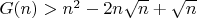$G(n) > n^2 - 2n \sqrt{n} + \sqrt{n} − 2$G(47)>1569Лучшая известная линейка Голомба =1804;Конечно есть куда отжигать. Но гложут меня смутные сомнения...Re: Al Zimmermann - Sums and Products25/08/12
160
Germany
 dimkadimon в сообщении #1083151 писал(а):Возможно самый лучший шанс это N=47. Это самое маленькое решение для которого не известна оптимальная линейка.All known optimal Golomb Rulers from N= 17 to 27 can be derived from an affine or projective plane construction. And in my optinion the probability that there exists some irregular optimal ruler for larger N gets even smaller with increasing N. So it is highly improbable that there is a better solution for N=47.Re: Al Zimmermann - Sums and Products13/12/15

91
 Herbert Kociemba в сообщении #1080806 писал(а):I did not examine the cases with prime p a bit larger than the problem size N yet (for example for N=1009 also p=1013 and p=1019 should be examined), but I am quite sure that all the top solutions for the larger N can be found in this way.Программа conapp для prime N использует только prime p=N. Программу conapp можно модифицировать для prime p>N?Re: Al Zimmermann - Sums and Products
 Заслуженный участник19/12/10
1546
 Последний раз редактировалось whitefox 21.12.2015, 16:03, всего редактировалось 1 раз. Можно, затем выбросите из полученной линейки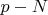$p-N$ лишних элементов (голомбовость при этом сохраняется).Re: Al Zimmermann - Sums and Products25/08/12
160
Germany
 Последний раз редактировалось Herbert Kociemba 21.12.2015, 19:47, всего редактировалось 1 раз. I think it is easier to write an own program to generate a cyclic difference set (CDS) compared to try to modify an existing program.For the contest we only need the case that the order is prime p, not the power of a prime p^k. Then we can use a linear recurrence of order 2 for the affine type and a linear recurrence of order 3 for the projective type to get some CDS from which all other CDS can be derived by shifts and multiplications.http://www.inference.phy.cam.ac.uk/cds/part11.htm andhttp://www.inference.phy.cam.ac.uk/cds/part12.htmis really all you need on a basic level. No need for the theory of finite fields at all - I really like these pages.Re: Al Zimmermann - Sums and Products21/02/10
1594
Екатеринбург
 Herbert Kociemba в сообщении #1084487 писал(а): cyclic difference set (CDS)А если так?https://en.wikipedia.org/wiki/Sparse_rulerЦитата:Wichmann rulers W(r,s) = 1^r, r+1, (2r+1)^r, (4r+3)^s, (2r+2)^(r+1), 1^r, where a^b represents b segments of length aУдаляя лишние числа, получаем линейку Голомба.Re: Al Zimmermann - Sums and Products25/08/12
160
Germany
 Последний раз редактировалось Herbert Kociemba 21.12.2015, 23:25, всего редактировалось 1 раз. Wichmann Rulers are useless since there are extremely many differences which are the same. This will not lead to good Golomb rulers.Re: Al Zimmermann - Sums and Products13/12/15

91
 Herbert Kociemba в сообщении #1081762 писал(а):Thank you! It took me really a long time to put all the pieces together. Now where the program is working I estimate it takes only a few hours to recompute the "best" solution for all 30 problems.Один из участников конкурса (россиянин), набравший 30 баллов, опубликовал в дискуссионной группе идею решения задачи в общих чертах. Как я понял, у него на случай n=5003 ушел всего один час. Т.к. остальные случаи требуют гораздо меньшего времени, то действительно задачу можно прогнать за несколько часов.Re: Al Zimmermann - Sums and Products13/12/15

91
 Herbert Kociemba в сообщении #1084487 писал(а):I think it is easier to write an own program to generate a cyclic difference set (CDS) compared to try to modify an existing program.For the contest we only need the case that the order is prime p, not the power of a prime p^k. Then we can use a linear recurrence of order 2 for the affine type and a linear recurrence of order 3 for the projective type to get some CDS from which all other CDS can be derived by shifts and multiplications.http://www.inference.phy.cam.ac.uk/cds/part11.htm andhttp://www.inference.phy.cam.ac.uk/cds/part12.htmis really all you need on a basic level. No need for the theory of finite fields at all - I really like these pages.conap и conapp выдают сразу два минимальных решения (для n=5003 это занимает около часа расчетного времени, если использовать несколько ядер с различными p для данного n). Далее по минимальному решению уже на одном ядре с помощью conap или conapp за время менее часа находятся два решения наиболее близких к минимальному. Полученного набора достаточно для решения задачи. Программы conap и conapp легко модифицируются.Re: Al Zimmermann - Sums and Products13/12/15

91
 Это я реализовал алгоритм, который описал Sigolaev Yurii в дискуссионной группе (ранее он его описал в общих чертах, и я не очень тогда его понял).Показать сообщения за: Все сообщения1 день7 дней2 недели1 месяц3 месяца6 месяцев1 год Поле сортировки АвторВремя размещенияЗаголовок по возрастаниюпо убыванию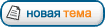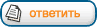Страница 11 из 11 [ Сообщений: 165 ] На страницу Пред.  1 ... 7, 8, 9, 10, 11

Модераторы: Karan, maxal, Toucan, PAV, Супермодераторы

#### Кто сейчас на конференции

Сейчас этот форум просматривают: нет зарегистрированных пользователей

 Вы не можете начинать темыВы не можете отвечать на сообщенияВы не можете редактировать свои сообщенияВы не можете удалять свои сообщенияВы не можете добавлять вложения

 Найти: